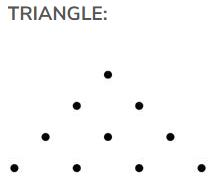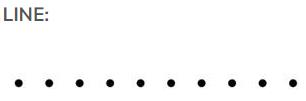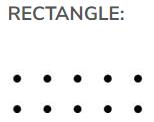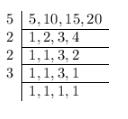Courses

## 20 Questions MCQ Test Mathematics (Maths) Class 6 | Olympiad Test: Whole Numbers

Description
This mock test of Olympiad Test: Whole Numbers for Class 6 helps you for every Class 6 entrance exam. This contains 20 Multiple Choice Questions for Class 6 Olympiad Test: Whole Numbers (mcq) to study with solutions a complete question bank. The solved questions answers in this Olympiad Test: Whole Numbers quiz give you a good mix of easy questions and tough questions. Class 6 students definitely take this Olympiad Test: Whole Numbers exercise for a better result in the exam. You can find other Olympiad Test: Whole Numbers extra questions, long questions & short questions for Class 6 on EduRev as well by searching above.
QUESTION: 1

### Which of the following is not true

Solution:

According to BODMAS rule, multiplication is done forst then addition.
So according to this, LHS = 7+ 8 x 9 = 7+72 = 79

But RHS =  (7 + 8) x(7+ 9) = (15) x (16) = 240

Hence Option C is incorrect

QUESTION: 2

### By using dot (•) patterns, which of the following numbers can be arranged in all the three ways namely a line, a triangle and a rectangle?

Solution:

A triangle can’t be made by 3 dots, 11 dots or 12 dots and a rectangle can’t be made by 11 dots, hence option A, C and D are incorrect.

A line, a triangle and a rectangle can be drawn by 10 dots as shown,QUESTION: 3

### Which of the following statements is not true?

Solution:
QUESTION: 4

Which of the following statements is not true?

Solution:

0 can not be divided by any number and 0 can not divide any number.
so, 0÷0=0 is not true

QUESTION: 5

The predecessor of 1 lakh is

Solution:

Predecessor is the term just before the given term

So 100000 - 1 = 99999

QUESTION: 6

The successor of 1 million is

Solution:
QUESTION: 7

Number of even numbers between 58 and 80 is

Solution:

Starting from 60, we have 60, 62, 64 .... upto 78 a total of 10 even numbers

QUESTION: 8

Sum of the number of primes between 16 to 80 and 90 to 100 is

Solution:

Prime numbers between 16 and 80 are

17 , 19 , 23 ,29 , 31 , 37 , 41 , 43 , 47 , 53 ,

59 , 61 , 67 , 71 , 73 , 79 ,

Number of primes between 16 and 80 = 16

Prime number between 90 and 100 is 97

Number of prime between 90 and 100 = 1

Therefore ,

Sum of the number of prime between 16 to 80

and 90 to 100 = 16 + 1 = 17

QUESTION: 9

Which of the following statements is not true?

Solution:
QUESTION: 10

The number of distinct prime factors of the largest 4-digit number is

Solution:

Largest 4 digit number = 9999

Prime Factorization of 9999 = 3²*11*101

Distinct prime factors of 9999 = 3, 11 and 101

Therefore, the total number of distinct prime factors of 9999 is 3 (i.e. 3, 11 and 101).

QUESTION: 11

The number of distinct prime factors of the smallest 5-digit number is

Solution:

Option A is right
The smallest 5− digit number =1000.
Now, we find the factor of 10000
∴10000=2×2×2×2×5×5×5×5
Therefore distinct prime factors of 10000 are 2 and 5,
Hence, 2 distinct prime factors.

QUESTION: 12

If the number 7254*98 is divisible by 22, the digit at * is

Solution:

7254698÷22=329759

QUESTION: 13

The largest number which always divides the sum of any pair of consecutive odd numbers is

Solution:
QUESTION: 14

A number is divisible by 5 and 6. It may not be divisible by

Solution:

Take the LCM of 5 and 6 is 30.

5 = 5×1

6 = 3×2×1

LCM of 5 and 6 is

5×1×3×2×1 = 30

And 30 is divisible by 10,15 and 30 but not by 60.

QUESTION: 15

The sum of the prime factors of 1729 is

Solution:

Prime factorization calculator of 1729

Positive Integer factors of 1729 = 7, 13, 91, 19, 1729 divided by 7, 13, 19, gives no remainder. They are integers and prime numbers of 1729, they are also called composite number.

So, on adding 7, 13, 19, we get the sum = 39.

QUESTION: 16

The greatest number which always divides the product of the predecessor and successor of an odd natural number other than 1, Is

Solution:

3,5,7,9 and so one are other than 1 odd natural number.
Now, the predecessor and successor of 3 are 2 and 4 respectively. The product is ⇒2×4=8
Similarly, the predecessor and successor of 5 are 4 and 6 respectively. The product is ⇒4×6=24 and so on.
Thus, the above shows that greatest number which always divides the product of the predecessor and successor of an odd natural other than 1 is 4

QUESTION: 17

The number of common prime factors of 75, 60, 105 is

Solution:

75 = 3 × 5 × 5

60 = 2 × 2 × 3 × 5

105 = 3 × 5 × 7

Therefore, common prime factors of 75, 60 and 105 are 3 and 5 that is 2 numbers are common.

QUESTION: 18

Which of the following pairs is not coprime?

Solution:
QUESTION: 19

Which of the following numbers is divisible by 11?

Solution:
QUESTION: 20

LCM of 10, 15 and 20 is

Solution:

LCM of 5 ,10, 15, 20 :-Hence, LCM of 5, 10, 15 and 20 is :-
5 x 2 x 2 x 3 = 60.